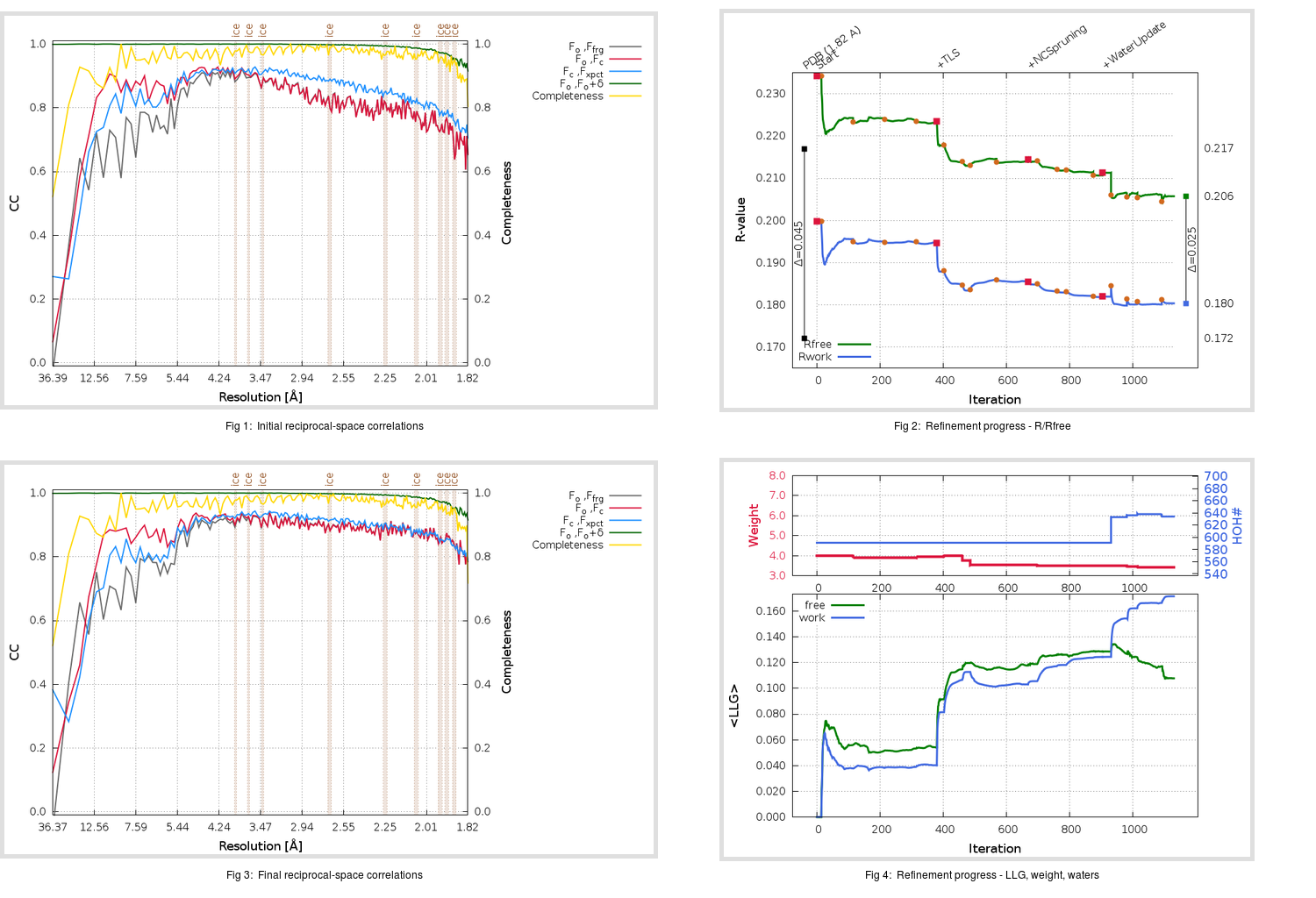Content:

```    Diffraction limits & principal axes of ellipsoid fitted to diffraction cut-off surface:
1.810         0.8802   0.0000   0.4745       0.931 a* + 0.364 c*
1.782         0.0000   1.0000   0.0000       b*
1.797        -0.4745   0.0000   0.8802      -0.355 a* + 0.935 c*
```

## Deposited

` `
 Date deposited Date data collection Resolution R, Rfree 20060427 20041117 1.82 0.1700 0.2170

Molprobity (CCP4 7.0 version) summary:

```Ramachandran outliers =   0.50 %
favored =  97.48 %
Rotamer outliers      =   4.07 %
C-beta deviations     =     7
Clashscore            =   7.69
RMS(bonds)            =   0.0196
RMS(angles)           =   1.76
MolProbity score      =   1.99
Resolution            =   1.82
R-work                =   0.1700
R-free                =   0.2170
```

```Number of waters      =   591

<B> (all atoms) =   30.95 ( sd =    7.08 ) for       5354 non-hydrogen atoms
<B>   (protein) =   30.09 ( sd =    6.17 ) for       4751 non-hydrogen atoms
<B>     (water) =   37.81 ( sd =    9.17 ) for        591 non-hydrogen atoms
<B>    (others) =   24.09 ( sd =   12.90 ) for         12 non-hydrogen atoms

B min/max       (all non-hydrogen atoms) =   13.82 /   65.53
B min/max   (protein non-hydrogen atoms) =   13.82 /   56.06
B min/max     (water non-hydrogen atoms) =   14.41 /   65.53
B min/max     (other non-hydrogen atoms) =   16.89 /   34.49
```

## BUSTER (re-)refinement

` `

Molprobity (CCP4 7.0 version) summary:

```Ramachandran outliers =   0.17 %
favored =  97.65 %
Rotamer outliers      =   3.70 %
C-beta deviations     =     6
Clashscore            =   3.37
RMS(bonds)            =   0.0115
RMS(angles)           =   1.60
MolProbity score      =   1.64
Resolution            =   1.82
R-work                =   0.1804
R-free                =   0.2057
```

```Number of waters      =   634

<B> (all atoms) =   28.46 ( sd =   11.10 ) for       5397 non-hydrogen atoms
<B>   (protein) =   26.58 ( sd =    9.44 ) for       4751 non-hydrogen atoms
<B>     (water) =   42.20 ( sd =   12.33 ) for        634 non-hydrogen atoms
<B>    (others) =   35.93 ( sd =   18.78 ) for         12 non-hydrogen atoms

B min/max       (all non-hydrogen atoms) =   11.77 /  123.96
B min/max   (protein non-hydrogen atoms) =   11.77 /   77.58
B min/max     (water non-hydrogen atoms) =   13.99 /  123.96
B min/max     (other non-hydrogen atoms) =   34.42 /   38.36
```

Refinement progression:Results:

` `
 File Remark 2GT7_aB_refine.01_04_refine.pdb.gz exact refinement commands are in header 2GT7_aB_refine.01_04_refine.mtz.gz including original deposited data and several re-refinement map coefficients 2GT7_aB_refine.01_04_BUSTER_model.cif.gz including any non-standard compound restraints 2GT7_aB_refine.01_04_BUSTER_refln.cif.gz